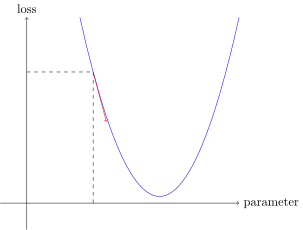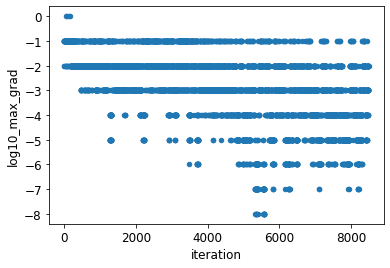# Why NN gradients are less than 1?

I have a guess, probably incorrect, regarding the behavior of gradients.
In the most first iteration of a NN, we just guessing our weights. Looking at some specific weight it should be pretty far from its final value. I expect the most first gradient of this weight must be pretty big, atleast bigger than 1. For example, like in Jeremy’s pictureWe see here the slope is bigger than 45 degrees -> the gradient is more than 1.

I want to explore it. I took 3 or 4 different models from the fastbook courses and still can’t find gradients (absolute values) more than 1.
For example

``````def abs_10_power(x):
return (x.abs() + (1e-30)).log10().flatten().numpy().astype(int)

def __init__(self): super().__init__(is_forward=False)
def before_fit(self):
super().before_fit()

def hook(self, m, i, o):

path = untar_data(URLs.MNIST_SAMPLE)
Path.BASE_PATH = path
learn = cnn_learner(dls, resnet18, pretrained=False,
loss_func=F.cross_entropy, metrics=accuracy)

learn.fit_one_cycle(1, 0.1, cbs=gcb)

.reset_index().rename(columns={'index': 'iteration'})\
``````Hi Sergey,

The parabola is just a particular example to illustrate SGD. When you go further from the minimum, the slope increases for a parabola, but that may not the case in general for the loss vs. weights function. I am really not sure - it would depend whether the loss function’s slope is bounded. It’s likely that for any we commonly use that the slope increases indefinitely when you get far from the minimum. What we actually rely on in SGD, though, is that the gradient gets smaller as we approach a local minimum. That does not mean that the opposite must necessarily be true.

I am delighted that you investigated your question with an actual experiment. A couple of comments:

• There is no reason to test against the number one. The scale of the gradient gets determined by the architecture and the loss function. (For example, when you put a factor in front of the loss function, it scales the gradient.) That’s why different models need different learning rates.

• So you would really want to test whether the gradient gets arbitrarily big as you move farther away. If you look at the gradients along a training path that already starts from a reasonable weight initialization, I doubt you will see any large values.

• A possibly more general way to measure the gradient is to take its norm (length) rather than the maximum abs() component.

• To observe some huge gradient values, try setting the learning rate too high. To be more intentional, you might create a loss function that rewards large losses, and train!

I hope these angles help. I am interested, theoretically, in knowing whether gradients get arbitrarily large toward the edges of weight space, so please post what you discover.In my assumptions about gradients and the underlying parameter-loss surface, I take the next picture in my mind from lesson 14 of the fastbook course

So here the only option to get max-gradients less than 1 in the whole process of learning, for all iterations, is to get into some of the local minimums. I don’t think this is a likely case.